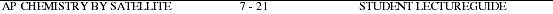###Go to Main Index

AP Chemistry by Satellite Lectureguide
Student Edition
Electronic Structure of Atoms: Trends and Periods
Chapter 7

Objectives

Following your study of this chapter, you should be able to

1. explain the differences between hydrogen-like (1-electron) orbitals and the atomic orbitals in a multi-electron atoms.
2. define the terms effective nuclear charge and screening effect and explain how the energy of the atomic orbitals are effected by the presence of more than one electron.
3. explain how the energies of the hydrogen-like (1-electron) orbitals change in a multi- electron atom.
4. describe the electron spin quantum number.
5. define and apply the Pauli exclusion principle to the process of building up a multi- electron atom.
6. list the four quantum numbers associated with each electron in multi-electron atoms and write the electron configuration for any element in the periodic table.
7. define and apply Hund's rule.
8. define valence electrons and core electrons and distinguish between them for any element in the periodic table.
9. identify the sections of the periodic table that contain s orbital, p orbital, d orbital and f orbital valence electrons.
10. identify electron configurations for elements from these regions of the periodic table: alkali metals, alkaline earth metals, transition metals, chalcogen, halogen, noble gas, lanthanide and actinides.
11. describe the trend in atomic radii among elements within groups and periods of the periodic table.
12. describe the trend in ionization energy among elements within groups and periods of the periodic table.
13. describe the trend in electron affinity among elements within groups and periods of the periodic table.
14. describe the observed trends in reactivity, atomic radii, ionization energy, and electron affinity for the alkali metal group.
15. compare and contrast properties of elements in the A group and elements in the B group.1. Explain the difference in the shapes of the s, p, d and f orbitals in a multi-electron atom compared to the s, p, d and f orbitals in the hydrogen atom.2. Define the terms effective nuclear charge and screening effect. To what extent do electrons in the same subshell screen each other from the nuclear charge. To what extent do core electrons screen valence electrons from the nucleus?3a. Draw an energy level diagram for the Bohr model of the hydrogen atom. Include only energy levels one through four.

b) Using the same approximate scale, draw an energy level diagram for the quantum mechanical model of a multi-electron atom.

c) Explain the differences between the two diagrams3d. Explain how adding electrons affects the degeneracy of energy levels.4. Briefly describe the spin quantum number and how it is related to the other three quantum numbers.5a. State the Pauli exclusion principle and illustrate using sets of four quantum numbers.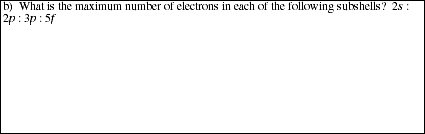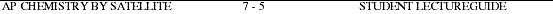6a. Prepare a list of all possible sets of quantum numbers for an electron in the n = 1, 2, and 3 levels. For each set of quantum numbers indicate the subshell designation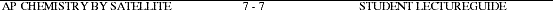7a. Define Hund's rule.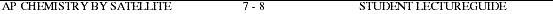8. Using the periodic table shown below indicate general electron configuration and the number of electrons in the valence shells for elements in each of the shaded groups.9. Use the blank periodic table below to mark the sections of the periodic table that contain elements having valence electrons in s orbitals, p orbitals, d orbitals and f orbitals.10a. Correlate group and period designations in the periodic table to the sections of the periodic table having valence electrons in s orbitals, p orbitals, d orbitals and f orbitals.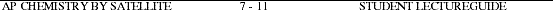11a. Use the blank periodic table below to display the general trends in atomic radii for elements within both groups and periods (rows). Explain the observed trends in terms of electronic screening effects and the populations of the various energy levels.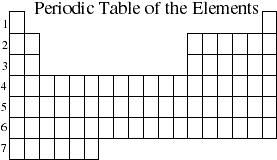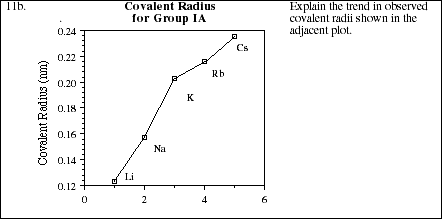12a. Use the blank periodic table below to display the general trends in ionization energy for elements within both groups and periods (rows). Explain the observed trends in terms of electronic screening effects and the populations of the various energy levels.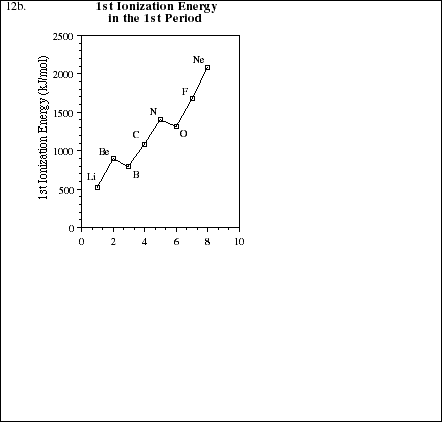13. Use the blank periodic table below to display the general trends in electron affinity for elements within both groups and periods (rows). Explain the observed trends in terms of electronic screening effects and the populations of the various energy levels.14. Describe the observed trends in reactivity, atomic radii, ionization energy, and electron affinity within the alkali metal group.15. Compare atomic radii, ionization energy, and electron affinity between corresponding members of the alkali metal group (IA) and the Group IB metals.Problem Set #11
AP Chemistry by Satellite

ALL work must be shown in all problems for full credit.

PS11.1. Of the set of four quantum numbers, n, l, ml, ms , which pair determines the energy of an electron in an orbital in a many electron atom?PS11.2. Order the following orbitals from highest to lowest energy.

4s, 2p, 4d, 3p, 4fPS11.3. Explain the term degenerate, as it relates to orbitals, and explain how adding electrons to a set of degenerate orbitals differs from adding electrons to orbitals which are not degenerate.PS11.4. List the possible values of the four quantum numbers for each electron of the valence electrons in Br.PS11.5. Draw the orbital diagrams for S and Mo.PS11.6. Write the electron configuration for Mg, Kr, Rh, Pt and Bi. Use the periodic table.PS11.7. Briefly explain each of the following statements.

a) The atomic radius of Mg is smaller than Ba.b) The ionization energy of S is lower than P.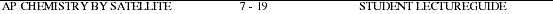PS11.7c) The energy required to remove the fourth electron in Al is significantly larger than the third electron.d) The energy change associated with gaining an electron, the electron affinity, is positive for some atoms and negative for others.PS11.8. Predict the products of the following reactions.

a) A sample of potassium is added to water at 25 ºC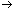b) Mg(s) + S(s)c) Sodium solid is dropped into a sample of bromined) Ba(s) + O2(g)Microcomputer softwareIntroduction to General Chemistry by Stan Smith, Ruth Chabay and Elizabeth Kean
Drill-and-practice software
\$500 (10-disk set)

Falcon Software
P.O Box 200
Wentworth, NH 03282
1-603-764-5788

Diskette #3 Chemical Formulas and Equations
Diskette #6 ChemazeComputer Aided Instruction for General Chemistry by William Butler & Raymond Hough Drill-and-practice software
\$40 (4-disk set)

John Wiley & Sons, Inc.
605 3rd Avenue
New York, NY 10158
(this software may not be available)

Diskette #1 Periodic Properties of the Elements
Diskette #2 Nomenclature and Oxidation Numbers